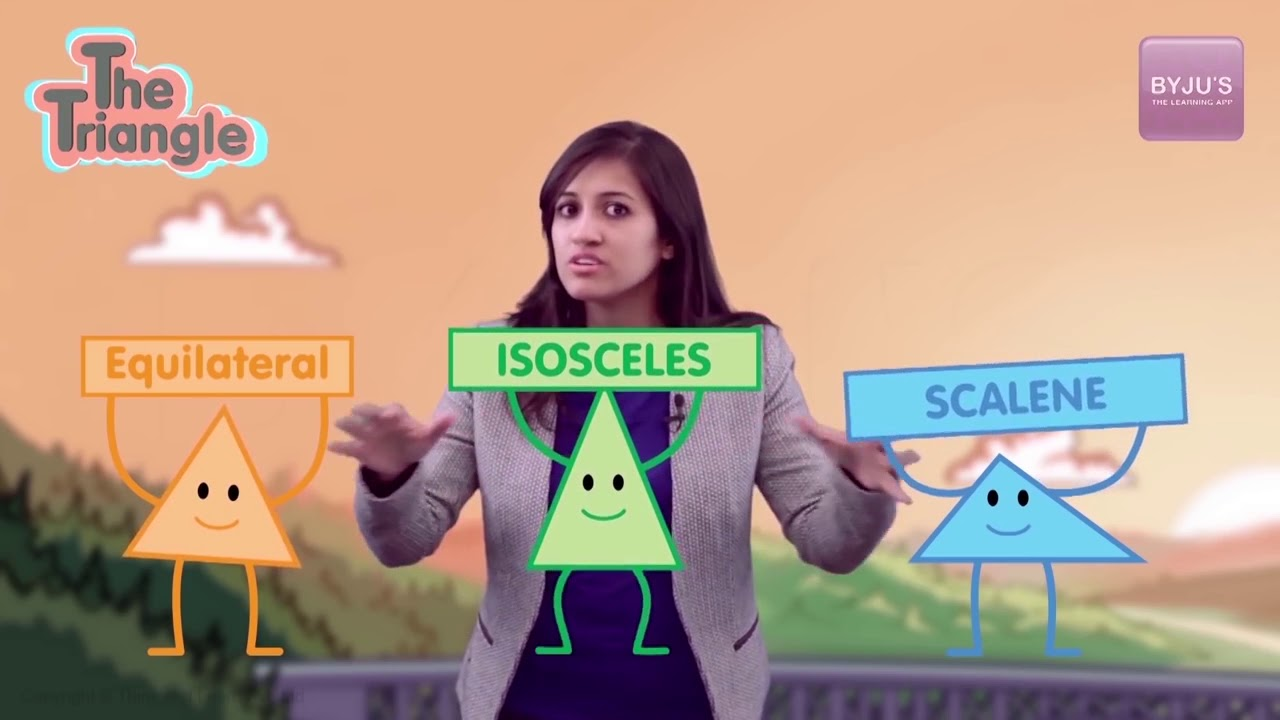# A triangle has side lengths of 3cm, 4cm, and, 6cm. Is it a right triangle explain?

A right-angled triangle is a type of triangle that has one of its angles equal to 90 degrees. The other two angles sum up to 90 degrees. The sides that include the right angle are perpendicular and the base of the triangle. The third side is called the hypotenuse, which is the longest side of all three sides. The side opposite to the right angle is the smallest side.

The three sides of the right triangle are related to each other. This relationship is explained by the Pythagoras theorem. According to this theorem, in a right triangle,

Hypotenuse2 = Perpendicular2 + Base

### Solution

Let us assume

Hypotenuse(h) = 6cm

Base(b) = 3cm

Altitude(a) = 4cm

According to the Pythagoras theorem,

Hypotenuse2 = Altitude2 + Base2

h2 = a2 + b2

⇒ h2 = 3² + 4²

= 9 +16 = 25

According to given hypotenuse h,

h= 62 = 36

The measures do not match

We cannot have a right triangle with the given measures.

Check out the video given below to know more about types of trianglesArticles to explore(0)(0)